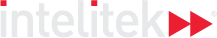### Course Overview

In the first course, the students develop a foundation for their future mathematical knowledge by studying number systems and working with
whole numbers, integers, and fractions, as well as measurements of different types, leading up to proportions, ratios and rates.

HOURS OF INSTRUCTION: 15

### Course Outline

• Symbols, Numbers, Numerals
• The Number Line and Number Systems: Natural Numbers, Integers
• Ratios, Rates and Proportion
• The Metric Decimal System
• Negative Numbers